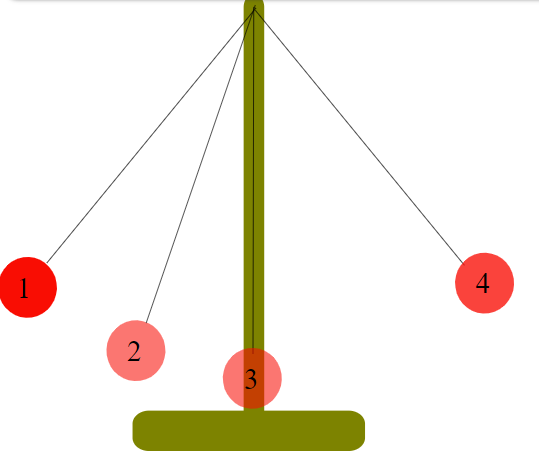# Kinetic and Potential Energy

$$\newcommand{\vecs}{\overset { \rightharpoonup} {\mathbf{#1}} }$$ $$\newcommand{\vecd}{\overset{-\!-\!\rightharpoonup}{\vphantom{a}\smash {#1}}}$$$$\newcommand{\id}{\mathrm{id}}$$ $$\newcommand{\Span}{\mathrm{span}}$$ $$\newcommand{\kernel}{\mathrm{null}\,}$$ $$\newcommand{\range}{\mathrm{range}\,}$$ $$\newcommand{\RealPart}{\mathrm{Re}}$$ $$\newcommand{\ImaginaryPart}{\mathrm{Im}}$$ $$\newcommand{\Argument}{\mathrm{Arg}}$$ $$\newcommand{\norm}{\| #1 \|}$$ $$\newcommand{\inner}{\langle #1, #2 \rangle}$$ $$\newcommand{\Span}{\mathrm{span}}$$ $$\newcommand{\id}{\mathrm{id}}$$ $$\newcommand{\Span}{\mathrm{span}}$$ $$\newcommand{\kernel}{\mathrm{null}\,}$$ $$\newcommand{\range}{\mathrm{range}\,}$$ $$\newcommand{\RealPart}{\mathrm{Re}}$$ $$\newcommand{\ImaginaryPart}{\mathrm{Im}}$$ $$\newcommand{\Argument}{\mathrm{Arg}}$$ $$\newcommand{\norm}{\| #1 \|}$$ $$\newcommand{\inner}{\langle #1, #2 \rangle}$$ $$\newcommand{\Span}{\mathrm{span}}$$$$\newcommand{\AA}{\unicode[.8,0]{x212B}}$$

Learning Objectives

• Define energy and its two main types listed below
• Describe the conservation of energy

You are probably familiar with these types of energy from a physics class. Energy is the capacity (ability, sort of) to do work. You have a sense of what work is from regular life, it's things that require effort. Energy and work have the same units. Kinetic energy is the energy that comes from motion. The equation for kinetic energy is

$KE=\frac{1}{2}mv^{2}$

where KE is kinetic energy, m is mass, and v is velocity. This definition should make sense: big things moving fast have the most energy, the most ability to shove other things or knock them over, etc. Potential energy is energy that comes from position and a force. For instance, gravitational potential energy is the energy that things have if they are high up. If they fall, their potential energy will turn into kinetic energy because they are accelerated by gravity. The equation for potential energy from gravity is

$PE=mgh$

where PE is potential energy, m is mass, g is the acceleration of gravity, and h is the height. This makes the units of energy very clear: mass x distance x acceleration, or force x distance, which comes to kg•m2s-2. In chemistry, the force that leads to potential energy is almost always the Coulomb force, not gravity. In this case, the potential energy from 2 charges near each other is

$PE=\frac{kQq}{d}$

where q and Q are the 2 charges, d is the distance between them, and k is a constant, 8.99 x 109 J•m•C-2. (Joules, J, are the SI unit of energy, and coulombs, C, are the SI unit of charge.) When the charges have the same sign, they repel and will accelerate away from each other if allowed to move; the potential energy has a positive sign. When the charges have the opposite sign, they attract each other and have negative potential energy. If they are allowed to get closer together, the potential energy will get more negative. If they are separated, d gets bigger and the potential energy approaches zero.A pendulum. The red ball is raised to Position 1 and released; it swings through Positions 2 and 3 to Position 4 on the other side.

Conservation of Energy

You probably learned about conservation of energy already in a physics class. For instance, if you have a pendulum as shown, at Position 1 the weight has some potential energy, but no kinetic energy. When you release the weight, the weight falls, moving through Position 2. At Position 2, some of the potential energy has been converted to kinetic energy. Finally, at Position 3, all the potential energy has been converted to kinetic energy. As it passes 3, the process is reversed, and kinetic energy is converted to potential energy. When the weight reaches Position 4, all the kinetic energy has been converted back to the same amount of potential energy it started with at 1. This is just one example of conservation of energy. It is a general observation that the amount of energy in the universe doesn't change, and the amount of energy in a particular system doesn't change unless there is a flow of energy in or out.